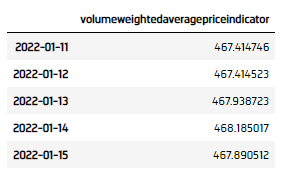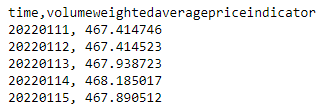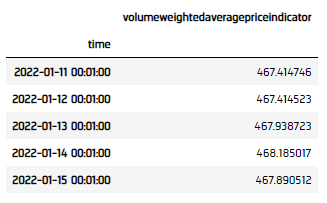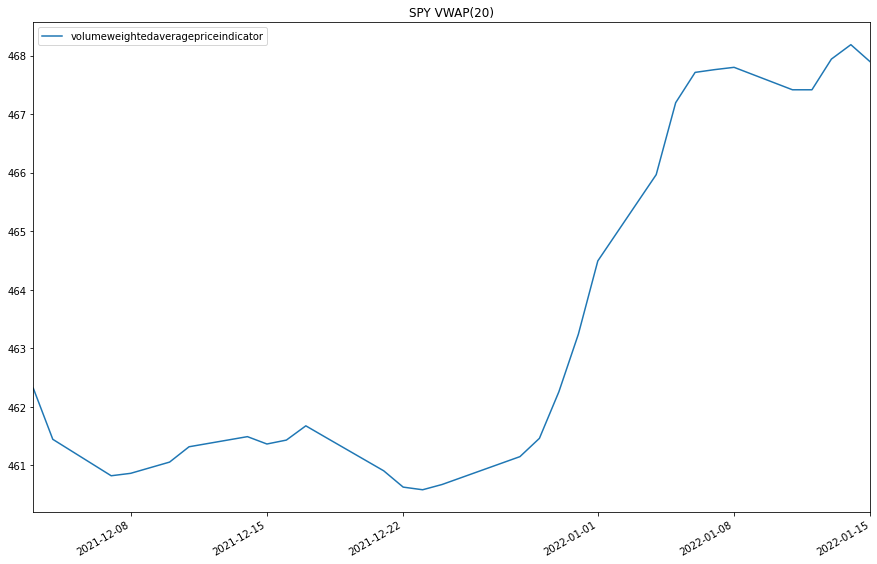# Indicators

### Introduction

This page explains how to create, update, and visualize LEAN TradeBar indicators.

### Create Subscriptions

You need to subscribe to some market data in order to calculate indicator values.

var qb = new QuantBook();
var symbol = qb.AddEquity("SPY").Symbol;
qb = QuantBook()
symbol = qb.AddEquity("SPY").Symbol

### Create Indicator Timeseries

You need to subscribe to some market data and create an indicator in order to calculate a timeseries of indicator values. In this example, use a 20-period VolumeWeightedAveragePriceIndicator indicator.

var vwap = new VolumeWeightedAveragePriceIndicator(20);
vwap = VolumeWeightedAveragePriceIndicator(20)

You can create the indicator timeseries with the Indicator helper method or you can manually create the timeseries.

#### Indicator Helper Method

To create an indicator timeseries with the helper method, call the Indicator method.

var vwapIndicator = qb.Indicator(vwap, symbol, 50, Resolution.Daily);
vwap_dataframe = qb.Indicator(vwap, symbol, 50, Resolution.Daily)#### Manually Create the Indicator Timeseries

Follow these steps to create an indicator timeseries:

1. Get some historical data.
2. var history = qb.History(symbol, 70, Resolution.Daily);
history = qb.History[TradeBar](symbol, 70, Resolution.Daily)
3. Create a RollingWindow for each attribute of the indicator to hold their values.
4. var time = new RollingWindow<DateTime>(50);
var window = new Dictionary<string, RollingWindow<decimal>>();
window["volumeweightedaveragepriceindicator"] = new RollingWindow<decimal>(50);

window = {}
window['time'] = RollingWindow[DateTime](50)
window['volumeweightedaveragepriceindicator'] = RollingWindow[float](50)

5. Attach a handler method to the indicator that updates the RollingWindow objects.
6. vwap.Updated += (sender, updated) =>
{
};
def UpdateVWAPWindow(sender: object, updated: IndicatorDataPoint) -> None:

vwap.Updated += UpdateVWAPWindow

When the indicator receives new data, the preceding handler method adds the new IndicatorDataPoint values into the respective RollingWindow.

7. Iterate through the historical market data and update the indicator.
8. foreach(var bar in history){
// Update the indicators with the whole TradeBar.
vwap.Update(bar);
}
for bar in history:
vwap.Update(bar)
9. Display the data.
10. Console.WriteLine($"time,{string.Join(',', window.Select(kvp => kvp.Key))}"); foreach (var i in Enumerable.Range(0, 5).Reverse()) { var data = string.Join(", ", window.Select(kvp => Math.Round(kvp.Value[i],6))); Console.WriteLine($"{time[i]:yyyyMMdd}, {data}");
}11. Populate a DataFrame with the data in the RollingWindow objects.
12. vwap_dataframe = pd.DataFrame(window).set_index('time')### Plot Indicators

Jupyter Notebooks don't currently support libraries to plot historical data, but we are working on adding the functionality. Until the functionality is added, use Python to plot TradeBar indicators.

Follow these steps to plot the indicator values:

1. Call the plot method.
2. vwap_indicator.plot(title="SPY VWAP(20)", figsize=(15, 10))
3. Show the plots.
4. plt.show()You can also see our Videos. You can also get in touch with us via Discord.